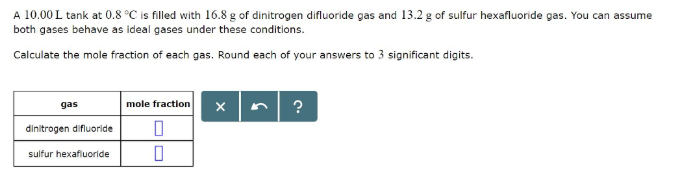# Problem: A 10.00 L tank at 0.8 degree C is filled with 16.8 g of dinitrogen difluoride gas and 13.2 g of sulfur hexafluoride gas. You can assume both gases behave as ideal gases under these conditions. Calculate the mole fraction of each gas. Round each of your answers to 3 significant digits.

###### FREE Expert Solution
80% (163 ratings)###### Problem Details

A 10.00 L tank at 0.8 degree C is filled with 16.8 g of dinitrogen difluoride gas and 13.2 g of sulfur hexafluoride gas. You can assume both gases behave as ideal gases under these conditions. Calculate the mole fraction of each gas. Round each of your answers to 3 significant digits.